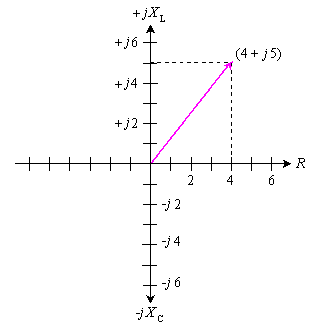Browse Definitions :
Definition

impedance

What is impedance?

Impedance, denoted Z, is an expression of the opposition that an electronic component, circuit, or system offers to alternating and/or direct electric current. Impedance is a vector (two-dimensional)quantity consisting of two independent scalar (one-dimensional) phenomena: resistance and reactance.

Resistance, denoted R, is a measure of the extent to which a substance opposes the movement of electrons among its atoms. The more easily the atoms give up and/or accept electrons, the lower the resistance, which is expressed in positive real number ohms. Resistance is observed with alternating current (AC) and also with direct current (DC). Examples of materials with low resistance, known as electrical conductors, include copper, silver, and gold. High-resistance substances are called insulators or dielectrics, and include materials such as polyethylene, mica, and glass. A material with an intermediate levels of resistance is classified as a semiconductor. Examples are silicon, germanium, and gallium arsenide.

Reactance, denoted X, is an expression of the extent to which an electronic component, circuit, or system stores and releases energy as the current and voltage fluctuate with each AC cycle. Reactance is expressed in imaginary number ohms. It is observed for AC, but not for DC. When AC passes through a component that contains reactance, energy might be stored and released in the form of a magnetic field, in which case the reactance is inductive (denoted +jXL); or energy might be stored and released in the form of an electric field, in which case the reactance is capacitive (denoted -jXC). Reactance is conventionally multiplied by the positive square root of -1, which is the unit imaginary number called the j operator, to express Z as a complex number of the form R + jXL (when the net reactance is inductive) or R - jXC (when the net reactance is capacitive).

The illustration shows a coordinate plane modified to denote complex-number impedances. Resistance appears on the horizontal axis, moving toward the right.(The left-hand half of this coordinate plane is not normally used because negative resistances are not encountered in common practice.)Inductive reactance appears on the positive imaginary axis, moving upward. Capacitive reactance is depicted on the negative imaginary axis, moving downward. As an example, a complex impedance consisting of 4 ohms of resistance and +j5 ohms of inductive reactance is denoted as a vector from the origin to the point on the plane corresponding to 4 + j5.In series circuits, resistances and reactances add together independently. Suppose a resistance of 100.00 ohms is connected in a series circuit with an inductance of 10.000 ?H.At 4.0000 MHz, the complex impedance is:

ZRL = R + jXL = 100.00 + j251.33

If a capacitor of 0.0010000 ?F is put in place of the inductor, the resulting complex impedance at 4.0000 MHz is:

ZRC = R - jXC = 100.00 - j39.789

If all three components are connected in series, then the reactances add, yielding a complex impedance of:

ZRLC = 100 + j251.33 - j39.789 = 100 + j211.5

This is the equivalent of a 100-ohm resistor in series with an inductor having +j211.5 ohms of reactance. At 4.0000 MHz, this reactance is presented by an inductance of 8.415 ?H, as determined by plugging the numbers into the formula for inductive reactance and working backwards.(See the definition of for this formula, and for the corresponding formula for capacitive reactance.)

Parallel RLC circuits are more complicated to analyze than are series circuits. To calculate the effects of capacitive and inductive reactance in parallel, the quantities are converted to inductive susceptance and capacitive susceptance. Susceptance is the reciprocal of reactance. Susceptance combines with conductance, which is the reciprocal of resistance, to form complex admittance, which is the reciprocal of complex impedance. Entire volumes have been devoted to the theoretical and practical aspects of resistance, conductance, reactance, susceptance, impedance, and admittance. An intermediate electronics text or reference book is recommended for further study.

This was last updated in December 2021

• ISO 31000 Risk Management

The ISO 31000 Risk Management framework is an international standard that provides businesses with guidelines and principles for ...

• pure risk

Pure risk refers to risks that are beyond human control and result in a loss or no loss with no possibility of financial gain.

• risk reporting

Risk reporting is a method of identifying risks tied to or potentially impacting an organization's business processes.

• What is risk mitigation?

Risk mitigation is a strategy to prepare for and lessen the effects of threats faced by a business.

• fault-tolerant

Fault-tolerant technology is a capability of a computer system, electronic system or network to deliver uninterrupted service, ...

• synchronous replication

Synchronous replication is the process of copying data over a storage area network, local area network or wide area network so ...

Close